# How to Count And Sum Cells by Color in Excel

This post will guide you how to count and sum cells by font color or cell color in Excel. How do I sum cell values by font color with VBA code in Excel. How to sum cells by color with formula in Excel.

## Sum Cells by Color with Filter feature

Assuming that you have a list of data in range A1:C6, which contain product name and its sale values, and the cells that contain sale values have been colored by different color. And you want to sum sale values by color in your worksheet. How to achieve it. You can use the filter command in combination with SUBTOTAL function to achieve the result. Just do the following steps:

#1 select the sale column in your range. And go to DATA tab, click Filter command under Sort & Filter group. And one filter icon will be added in the first cell of sales column.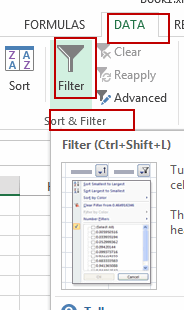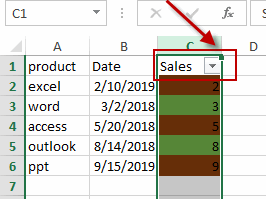#2 click filter icon on the Cell C1, and click Filter by Color, then select one color that you want to be filtered (such as: select green color as filtered color). Click Ok button.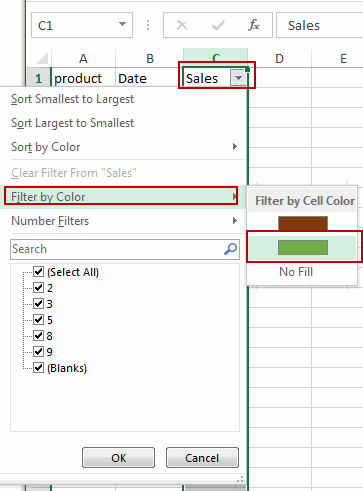#3 all cell will be filtered out by green color.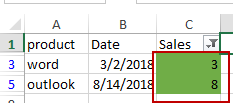#4 Type the following formula in a blank cell to sum cells by green color, and then press Enter key in your keyboard.

`=SUBTOTAL(9,A2:C6)`

You will see that cell values will be counted by green color only.

##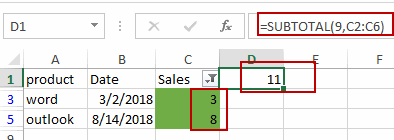Sum Cells by Color with VBA Code

You can also write a User Defined Function to achieve the result of summing cells by Cell color in Excel. Just do the following steps:

#1 open your excel workbook and then click on “Visual Basic” command under DEVELOPER Tab, or just press “ALT+F11” shortcut.#2 then the “Visual Basic Editor” window will appear.

#3 click “Insert” ->”Module” to create a new module.#4 paste the below VBA code into the code window. Then clicking “Save” button.

##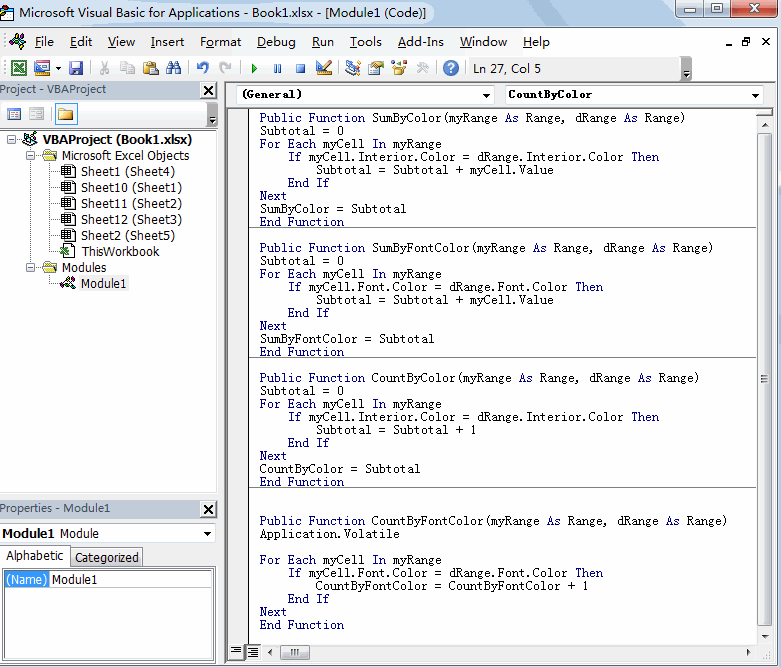```Public Function SumByColor(myRange As Range, dRange As Range)
Subtotal = 0
For Each myCell In myRange
If myCell.Interior.Color = dRange.Interior.Color Then
Subtotal = Subtotal + myCell.Value
End If
Next
SumByColor = Subtotal
End Function```

#5 back to the current worksheet, then type the following formula in a blank cell. press Enter key.

`=SumByColor(C2:C6,C3)`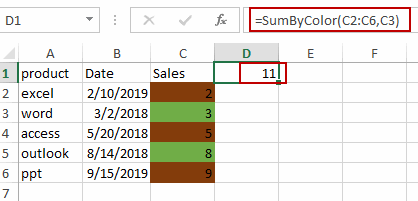## Sum Cells by Font Color with VBA Code

If you want to sum cells by Font color for in a given range of cells in Excel, you can also write a User Defined function to achieve the result. Just do the following steps:

#1 repeat the above steps 1-3.

#2 paste the below VBA code into the code window. Then clicking “Save” button.

```Public Function SumByFontColor(myRange As Range, dRange As Range)
Subtotal = 0
For Each myCell In myRange
If myCell.Font.Color = dRange.Font.Color Then
Subtotal = Subtotal + myCell.Value
End If
Next
SumByFontColor = Subtotal
End Function```

#3 back to the current worksheet, then type the following formula in a blank cell. press Enter key.

=SumByFontColor(C2:C6,C3)

## Count Cells by Color with VBA Code

If you only want to count cells by one color in your range of cells, you can use a User Defined Function to achieve the result. Just do the following steps:

#1 open your excel workbook and then click on “Visual Basic” command under DEVELOPER Tab, or just press “ALT+F11” shortcut.#2 then the “Visual Basic Editor” window will appear.

#3 click “Insert” ->”Module” to create a new module.#4 paste the below VBA code into the code window. Then clicking “Save” button.

```Public Function CountByColor(myRange As Range, dRange As Range)
Subtotal = 0
For Each myCell In myRange
If myCell.Interior.Color = dRange.Interior.Color Then
Subtotal = Subtotal + 1
End If
Next
CountByColor = Subtotal
End Function```

#5  back to the current worksheet, then type the following formula in a blank cell. press Enter key.

=CountByColor(C2:C6,C3)

## Count Cells by Font Color with VBA Code

If you want to count cells by Font Color, you can use the below User Defined function to achieve the result.

```Public Function CountByFontColor(myRange As Range, dRange As Range)
Application.Volatile

For Each myCell In myRange
If myCell.Font.Color = dRange.Font.Color Then
CountByFontColor = CountByFontColor + 1
End If
Next
End Function```

##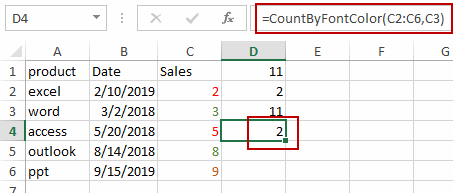Video: Count and Sum Cells by Color

### Related Functions

• Excel SUBTOTAL function
The Excel SUBTOTAL function returns the subtotal of the numbers in a list or database. The syntax of the SUBTOTAL function is as below:= SUBTOTAL (function_num, ref1, [ref2])….